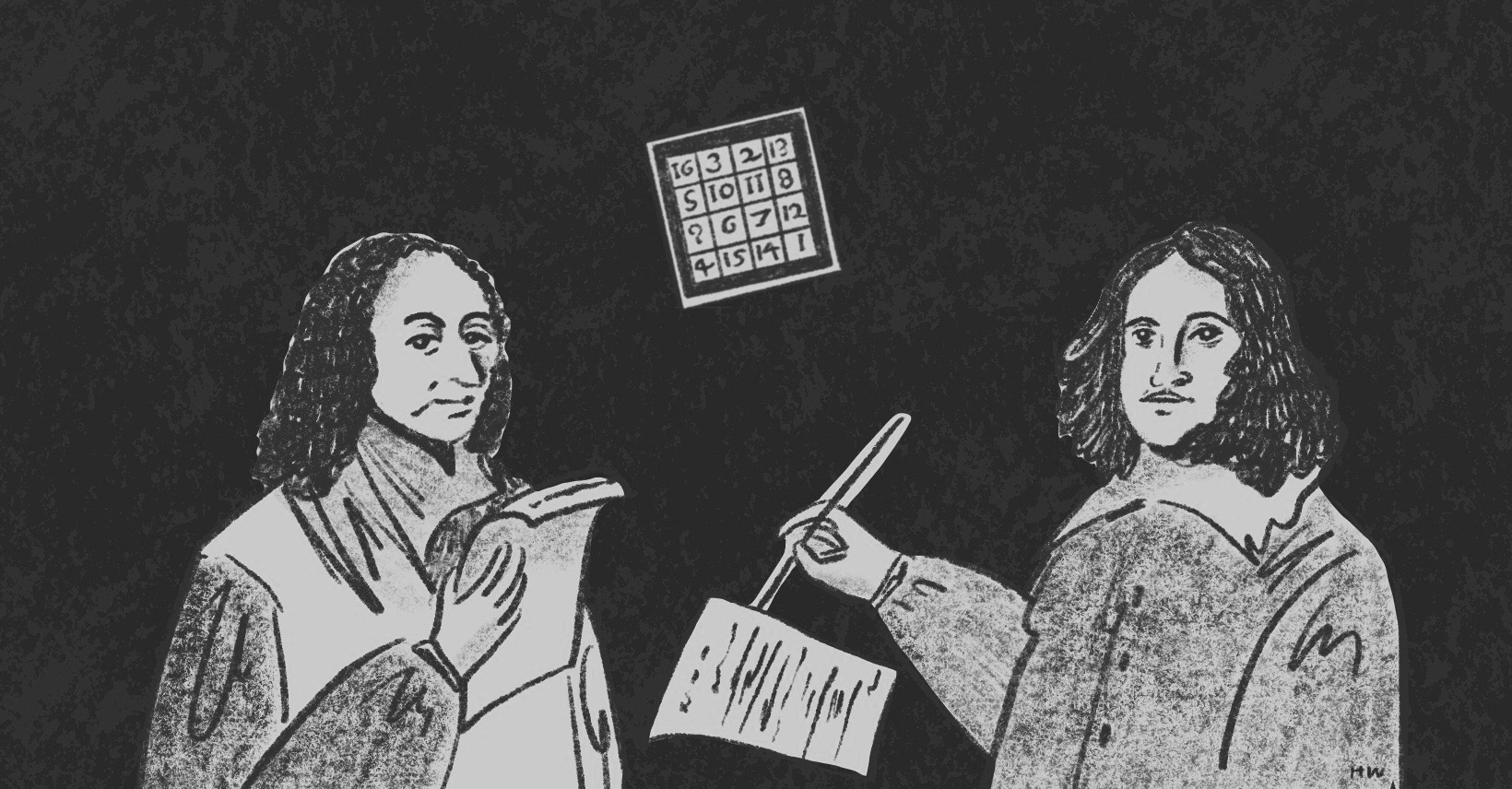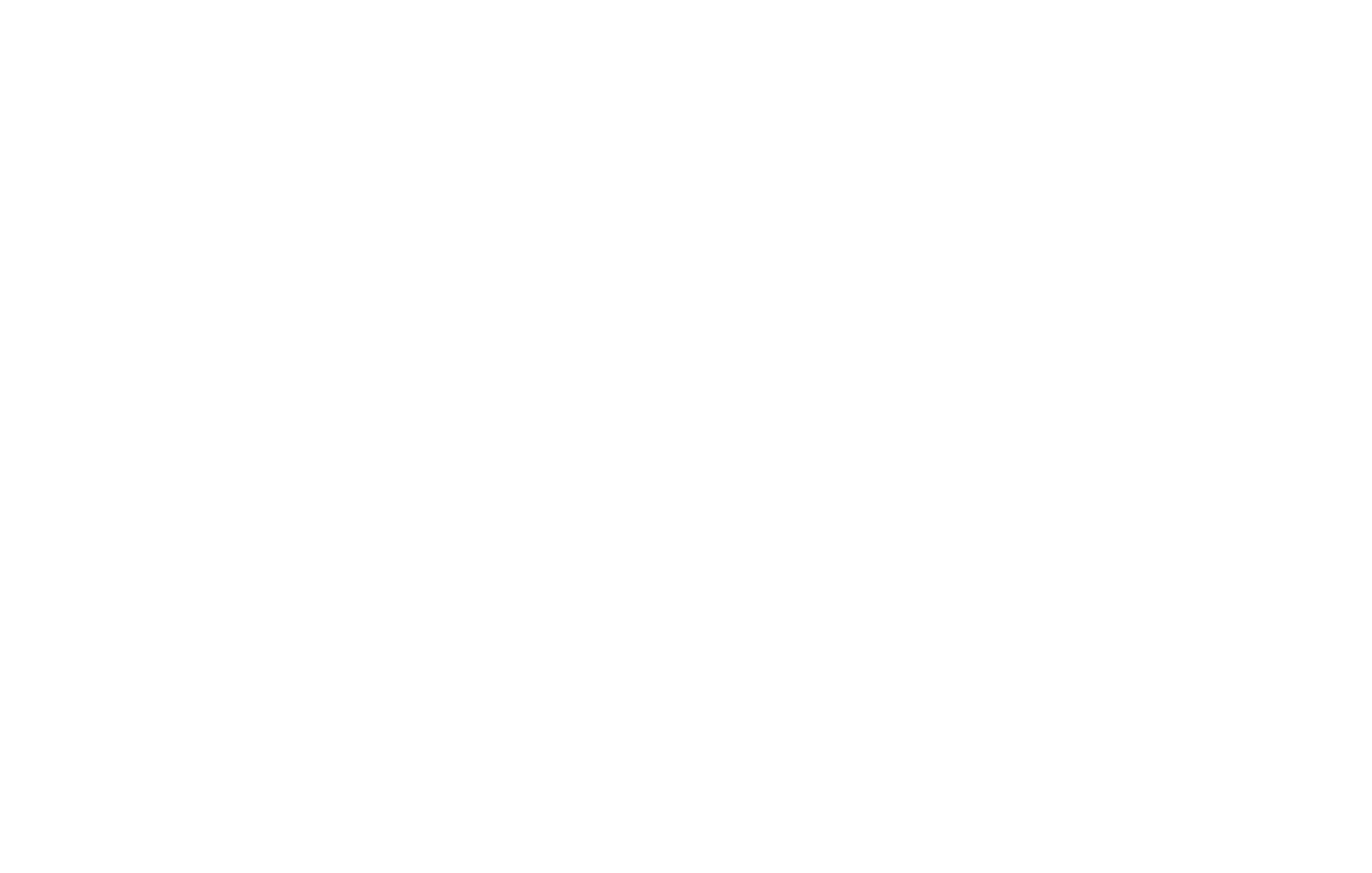×資料管理 統計分析 相關資源 巨人肩膀 語法索引 關於作者

## 簡介

• solve() -解方程式
• licence() -R版權頁
• demo() -展示可用指令
• help() -線上說明文件
• history() -輸入指令的歷史紀錄
• savehistory() -儲存指令歷史紀錄
• q() -關閉R程式

R所有操作都在R Console中完成，紅色游標：>是輸入指令的地方。

 > 2-3  -1 > 3*4  12 > 1+3^2  10 > (8-4)^(1/2)  2 > x=2 > y=10 > x^y  1024 

 > equation1<-c(2,3) > equation2<-c(1,-1) > C<-c(-4,3) > E<-rbind(equation1, equation2) > solve(E, C)  1 -2 

 > equation3<-c(2,2,1) > equation4<-c(5,3,2) > equation5<-c(3,3,1) > C2<-c(10,19,13) > E2<-rbind(equation1, equation2, equation3) > solve(E2, C2)  1 2 4 

R的指令由名稱+括弧所組成，括弧內可以空白，也可以自行輸入其他屬性。例如輸入licence()呼叫R的版本、demo()呼叫可以使用的指令、demo(graphics)呼叫繪圖功能。

 > licence() > demo() > demo(graphics) 

 > help() > help(getwd) > ??setwd 

 > history() > savehistory() > loadhistroy() 
 > q()